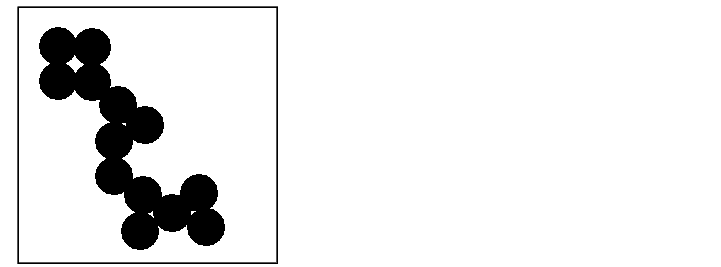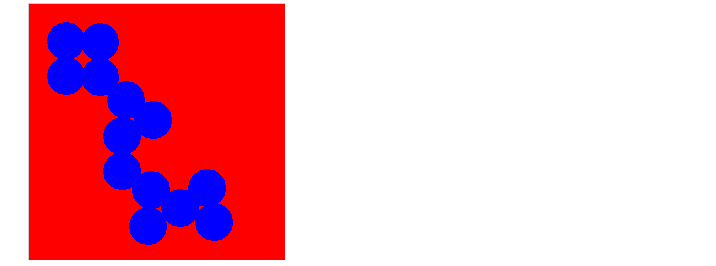Image Processing Toolbox User's GuideDisplaying Binary Images

In MATLAB, a binary image is of class `logical`. Binary images contain only 0's and 1's. Pixels with the value 0 are displayed as black; pixels with the value 1 are displayed as white.

 Note    For the toolbox to interpret the image as binary, it must be of class `logical`. Grayscale images that happen to contain only 0's and 1's are not binary images.

To display a binary image, using either `imshow` or `imtool`, specify the image matrix as an argument. For example, this code reads a binary image into the MATLAB workspace and then displays the image. This documentation uses the variable name `BW` to represent a binary image in the workspace

• ```BW = imread('circles.png');
imshow(BW)
```

or

• ```imtool(BW)```

Changing the Display Colors of a Binary Image

You might prefer to invert binary images when you display them, so that 0 values are displayed as white and 1 values are displayed as black. To do this, use the NOT (~) operator in MATLAB. (In this figure, a box is drawn around the image to show the image boundary.) For example:

• ```imshow(~BW)
```

or

• ```imtool(~BW)```

You can also display a binary image using the indexed image colormap syntax. For example, the following command specifies a two-row colormap that displays 0's as red and 1's as blue.

• ```imshow(BW,[1 0 0; 0 0 1])
```

or

• ```imtool(BW,[1 0 0; 0 0 1])```Displaying Grayscale Images Displaying Truecolor Images